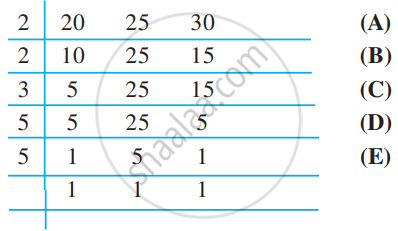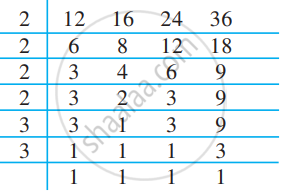# Lowest Common Multiple:

The Least Common Multiple of the given numbers is the smallest number that is divisible by each of the given numbers. To find the LCM of the given numbers, we write down the multiples of each of the given numbers and find the lowest of their common multiples.

### a) LCM by Prime Factorization Method:

Find the LCM of 40, 48, and 45.

The prime factorisations of 40, 48 and 45 are;
40= 2 × 2 × 2 × 5
48= 2 × 2 × 2 × 2 × 3
45= 3 × 3 × 5
The prime factor 2 appears the maximum number of four times in the prime factorisation of 48, the prime factor 3 occurs the maximum number of two times

in the prime factorisation of 45, The prime factor 5 appears one time in the prime factorisations of 40 and 45, we take it only once.

Therefore, required LCM = (2 × 2 × 2 × 2) × (3 × 3) × 5 = 720.

### b) LCM by Division Method:

Find the LCM of 20, 25 and 30.

We write the numbers as follows in a row:So, LCM = 2 × 2 × 3 × 5 × 5.

(A) Divide by the least prime number which divides at least one of the given numbers. Here, it is 2. The numbers like 25 are not divisible by 2 so they are written as such in the next row.

(B) Again divide by 2. Continue this till we have no multiples of 2.
(C) Divide by next prime number which is 3.
(D) Divide by next prime number which is 5.
(E) Again divide by 5.

#### Example

In a morning walk, three persons step off together. Their steps measure 80 cm, 85 cm, and 90 cm respectively. What is the minimum distance each should walk so that all can cover the same distance in complete steps?

The distance covered by each one of them is required to be the same as well as a minimum. The required minimum distance each should walk would be the lowest common multiple of the measures of their steps.

Thus, we find the LCM of 80, 85, and 90.

The LCM of 80, 85, and 90 is 12240.

The required minimum distance is 12240 cm.

#### Example

Find the least number which when divided by 12, 16, 24 and 36 leaves a remainder 7 in each case.

We first find the LCM of 12, 16, 24, and 36 as follows:Thus, LCM = 2 × 2 × 2 × 2 × 3 × 3 = 144

144 is the least number which when divided by the given numbers will leave remainder 0 in each case. But we need the least number that leaves remainder 7in each case.
Therefore, the required number is 7 more than 144.

The required least number = 144 + 7 = 151.

#### Example

Add: 17/28 + 11/35

Let us find the LCM of 28 and 35 in order to add the fractions.

LCM = 7 × 4 × 5 = 140

17/28 + 11/35 = (17 xx 5)/(28 xx 5) + (11 xx 4)/(35 xx 4) = (85 + 44)/140 = 129/140.

#### Example

On dividing a certain number by 8, 10, 12, 14 the remainder is always 3. Which is the smallest such number?

 2 8 10 12 14 2 4 5 6 7 2 5 3 7

Let us find the LCM of the given divisors.
LCM = 2 × 2 × 2 × 5 × 3 × 7 = 840.
To the LCM we add the remainder obtained every time.
Hence, that number = LCM + remainder = 840 + 3 = 843

#### Example

Shreyas, Shalaka, and Snehal start running from the same point on a circular track at the same time and complete one lap of the track in 16 minutes, 24 minutes, and 18 minutes respectively. What is the shortest period of time in which they will all reach the starting point together?

The number of minutes they will take to reach together will be a multiple of 16, 24, and 18.
16 = 2 × 2 × 2 × 2
24 = 2 × 2 × 2 × 3
18 = 2 × 3 × 3
LCM = 2 × 2 × 2 × 2 × 3 × 3 = 144.
They will come together in 144 minutes or 2 hours 24 minutes.

If you would like to contribute notes or other learning material, please submit them using the button below.

### Shaalaa.com

Word Problem On LCM Part - 1 [00:20:01]
S
0%